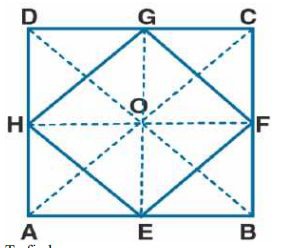# ML Aggarwal Solutions Class 9 Mathematics Solutions for Mid Point Theorem Exercise 11 in Chapter 11 - Mid Point Theorem

Show that the quadrilateral formed by joining the mid-points of the adjacent sides of a square, is also a

square.

It is given that

A square ABCD in which E, F, G and H are mid-points of AB, BC, CD and DA

Join EF, FG, GH and HETo find:

EFGH is a square

Construct AC and BD

Proof:

In Δ ACD

G and H are the mid-points of CD and AC

GH || AC and GH = ½ AC ….. (1)

In Δ ABC, E and F are the mid-points of AB and BC

EF || AC and EF = ½ AC …… (2)

Using both the equations

EF || GH and EF = GH = ½ AC ….. (3)

In the same way, we can prove that

EF || GH and EH = GF = ½ BD

We know that the diagonals of the square are equal

AC = BD

By dividing both sides by 2

½ AC = ½ BD ….. (4)

Using equation (3) and (4)

EF = GH = EH = GF …. (5)

Therefore, EFGH is a parallelogram

In Δ GOH and Δ GOF

OH = OF as the diagonals of a parallelogram bisect each other

OG = OG is common

Using equation (5)

GH = GF

Δ GOH ≅ Δ GOF (SSS axiom of congruency)

∠GOH = ∠GOF (c.p.c.t)

We know that

∠GOH + ∠GOF = 180^{\circ}

∠GOH + ∠GOH = 180^{\circ}

So we get

2 ∠GOH = 180^{\circ}

∠GOH = 180^{\circ} / 2=90^{\circ}

So the diagonals of a parallelogram ABCD bisect and perpendicular to each other

Hence, it is proved that EFGH is a square.

Related Questions
Exercises

Lido

Courses

Teachers

Book a Demo with us

Syllabus

Maths
CBSE
Maths
ICSE
Science
CBSE

Science
ICSE
English
CBSE
English
ICSE
Coding

Terms & Policies

Selina Question Bank

Maths
Physics
Biology

Allied Question Bank

Chemistry
Connect with us on social media!